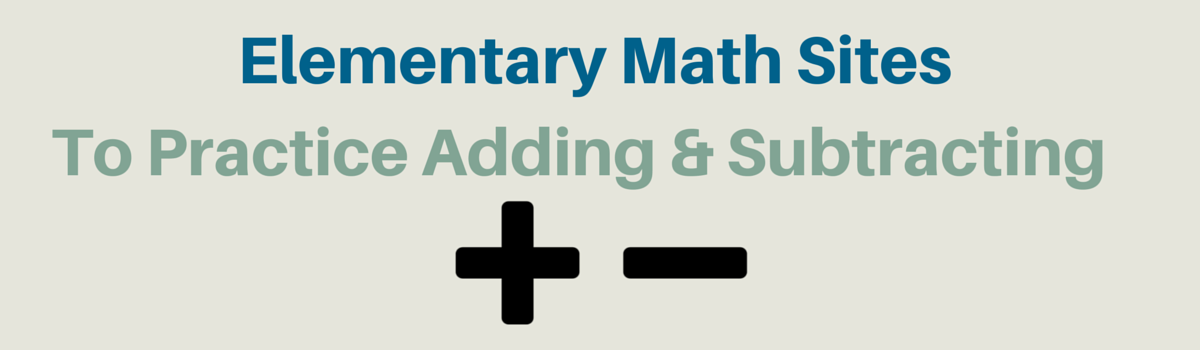Updated by Jon Samuelson on Nov 04, 2019REPORTJon Samuelson
Owner
18 items   10 followers   0 votes   5.77k views

# Elementary Math Websites To Practice Addition + Subtraction

A list developed by Cindy Lawrence, 3rd grade teacher in Texas. This list accompanies Unit 2 of the Third Grade Everyday Math Curriculum.

1

## Skater MathPlay Skater Math and other math games at hoodamath.com.

2Play Alien Addition and other math games at hoodamath.com.

3Jet Ski Addition is an educational multi-player racing game for addition. How quickly the student correctly answers the addition problems determines how quickly the jet ski will go. The student with the fastest rate of correct answers will win the race. Hits and misses are recorded and displayed at the end of the game, along with the student's rate. 1-4 players can play at once.

4

## Math Games: Fruit Shoot Addition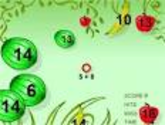Learn how to add the fun way with Fruit Shoot Addition math game.

5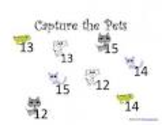6

## Math Games: Addition Wth Missing Numbers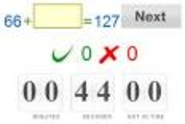Matching game teaches addition, solve for the missing number.

7

## BBC - KS2 Bitesize Maths - Addition : PlayMaths number exercise - Addition activity

8

## Math Games: Fruit Shoot Subtraction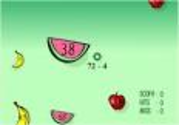Learn subtraction the fun way with Fruit Shoot Subtraction.

9

## Math Games: Popup Subtraction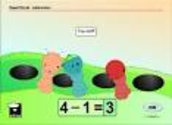Learn subtraction the fun way with this arcade style math game.

10

## Math Games: Fruit Shoot Number Line Subtraction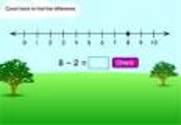Learn how to add the fun way with Fruit Shoot Number Line Subtraction math game.

11

## Math Games: Subtraction Wth Missing Numbers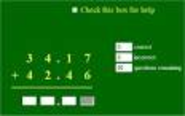Matching game teaches subtraction, solve for the missing number.

12

## BBC - Schools - Teachers - KS2 Maths - Subtraction activityAn animated KS2 activity for the Maths topic: Subtraction

13

## Subtracting Two-Digit Numbers Game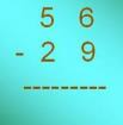Fun soccer math game about subtracting two-digit numbers without borrowing.

14

## Subtracting Money GameStudents everywhere love this fun online interactive game about subtracting money.

15

## ABCya! Math Man JUNIOR | ADDITION & SUBTRACTIONMath Man Jr. is an elementary activity to practice addition and subtraction math facts. Solve math problems and avoid the ghosts! Fun for children of all ages!

16

## War Pretzels | MrNussbaum.com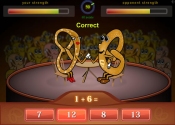17

## Bowling Pin Math | MrNussbaum.com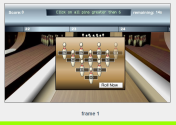18

## Mental Math Trainer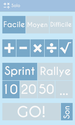Practice mental arithmetic online. No ads and no charge.